Test: Cubes and Dice- 2

# Test: Cubes and Dice- 2 - Class 10

Test Description

## 15 Questions MCQ Test Olympiad Preparation for Class 10 - Test: Cubes and Dice- 2

Test: Cubes and Dice- 2 for Class 10 2023 is part of Olympiad Preparation for Class 10 preparation. The Test: Cubes and Dice- 2 questions and answers have been prepared according to the Class 10 exam syllabus.The Test: Cubes and Dice- 2 MCQs are made for Class 10 2023 Exam. Find important definitions, questions, notes, meanings, examples, exercises, MCQs and online tests for Test: Cubes and Dice- 2 below.
Solutions of Test: Cubes and Dice- 2 questions in English are available as part of our Olympiad Preparation for Class 10 for Class 10 & Test: Cubes and Dice- 2 solutions in Hindi for Olympiad Preparation for Class 10 course. Download more important topics, notes, lectures and mock test series for Class 10 Exam by signing up for free. Attempt Test: Cubes and Dice- 2 | 15 questions in 30 minutes | Mock test for Class 10 preparation | Free important questions MCQ to study Olympiad Preparation for Class 10 for Class 10 Exam | Download free PDF with solutions
 1 Crore+ students have signed up on EduRev. Have you?
Test: Cubes and Dice- 2 - Question 1

### A cuboid shaped wooden block has 6 cm length, 4 cm breadth and 1 cm height. Two faces measuring 4 cm × 1 cm are coloured in black. Two faces measuring 6 cm × 1 cm are coloured in red. Two faces measuring 6 cm × 4 cm are coloured in green. The block is divided into 6 equal cubes of side 1 cm (from 6 cm side), 4 equal cubes of side 1 cm (from 4 cm side). How many cubes will remain if the cubes having black and green coloured are removed?

Detailed Solution for Test: Cubes and Dice- 2 - Question 1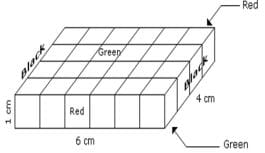Number of small cubes which are black and green is 8 in all.
Hence, the number of remaining cubes are = 24 – 8 = 16

Test: Cubes and Dice- 2 - Question 2

### A cuboid of dimensions (6 cm × 4 cm × 1 cm) is painted black on both the surfaces of dimensions ( 4 cm × 1 cm) green on the surfaces of dimensions (6 cm × 4 cm). and red on the surfaces of dimensions (6 cm × 1 cm). Now the block is divided into various smaller cubes of side 1 cm. each. The smaller cubes so obtained are separated. How many cubes will be formed?

Test: Cubes and Dice- 2 - Question 3

### A cuboid of dimensions (6 cm × 4 cm × 1 cm) is painted black on both the surfaces of dimensions ( 4 cm × 1 cm) green on the surfaces of dimensions (6 cm × 4 cm). and red on the surfaces of dimensions (6 cm × 1 cm). Now the block is divided into various smaller cubes of side 1 cm. each. The smaller cubes so obtained are separated. What should be the number opposite to 4?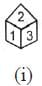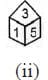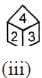Test: Cubes and Dice- 2 - Question 4

The figure (X) given below is the unfolded position of a cubical dice. In each of the following questions, this unfolded figure is followed by four different figures of dice. You have to select the figure which is identical to the figure (X).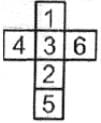Test: Cubes and Dice- 2 - Question 5

All the opposite faces of a big cube are coloured with red, black and green colours. After that, is cut it into 64 small equal cubes. In each of the following questions, select the correct option for the questions asked.

How many small cubes are there where one face is green and other one is either black or red?

Detailed Solution for Test: Cubes and Dice- 2 - Question 5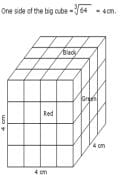Number of small cubes having one face green and the other one is either red or black = 8 × 2 = 16

Test: Cubes and Dice- 2 - Question 6

All the opposite faces of a big cube are coloured with red, black and green colours. After that, is cut it into 64 small equal cubes. In each of the following questions, select the correct option for the questions asked.

How many small cubes are there with 3 faces coloured?

Detailed Solution for Test: Cubes and Dice- 2 - Question 6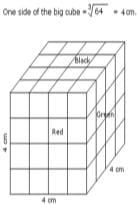Number of small cubes having three faces coloured = 1 at each corner = 1 × 8 = 8

Test: Cubes and Dice- 2 - Question 7

Which symbol will appear opposite to x?
(i)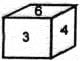(ii)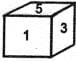Test: Cubes and Dice- 2 - Question 8

On the basis of the following figures, you have to tell which number will come in place of ‘?’
(i)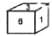(ii)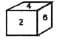(iii)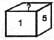Test: Cubes and Dice- 2 - Question 9

Choose from the alternatives, the boxes that will be formed when figure (X) is folded: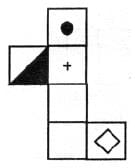Test: Cubes and Dice- 2 - Question 10

Choose from the alternatives, the boxes that will be formed when figure (X) is folded: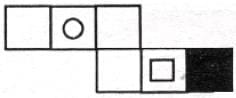Test: Cubes and Dice- 2 - Question 11

A cube is coloured orange on one face, pink on the opposite face, brown on one face and silver on a face adjacent to the brown face. The other two faces are left uncoloured. It is then cut into 125 smaller cubes of equal size. Now answer the following questions based on the above statements.

How many cubes have all the faces uncoloured?

Detailed Solution for Test: Cubes and Dice- 2 - Question 11

Now we know that 2 adjacent faces are not painted. We know for sure that all the cubes which are present in the center of the bigger cube, i.e., all the cubes that are not visible to human eye are not colored. Number of such cubes are : 3 ∗ 3 ∗ 3 = 27. But there are also some cubes which are visible to us on the sides which are left uncolored. Number of such cubes are : 4 ∗ 3 + 3 ∗ 3 = 21. Do not count the cubes on the uncolored edges twice while doing this calculation. So total uncolored cubes : 27 + 21 = 48
You can solve this in a simpler manner if you imagine that , uncolored cubes will be in the form of a 4 × 4 × 3 cube, which gives us 48 uncolored cubes.

Test: Cubes and Dice- 2 - Question 12

A cube is coloured orange on one face, pink on the opposite face, brown on one face and silver on a face adjacent to the brown face. The other two faces are left uncoloured. It is then cut into 125 smaller cubes of equal size. Now answer the following questions based on the above statements.

How many cubes are coloured orange on one face and have the remaining faces uncoloured?

Detailed Solution for Test: Cubes and Dice- 2 - Question 12

Only one face colored.

Test: Cubes and Dice- 2 - Question 13

A cube is painted red on two adjacent surfaces and black on the surfaces opposite to red surfaces and green on the remaining faces. Now the cube is cut into sixty four smaller cubes of equal size.

How many smaller cubes have only one surface painted?

Test: Cubes and Dice- 2 - Question 14

A cube is painted red on two adjacent surfaces and black on the surfaces opposite to red surfaces and green on the remaining faces. Now the cube is cut into sixty four smaller cubes of equal size.

How many smaller cubes have less than three surfaces painted?

Test: Cubes and Dice- 2 - Question 15

A cube is painted red on two adjacent surfaces and black on the surfaces opposite to red surfaces and green on the remaining faces. Now the cube is cut into sixty four smaller cubes of equal size.

How many smaller cubes with two surfaces painted have one face green and one of the adjacent faces black or red?

## Olympiad Preparation for Class 10

11 videos|36 docs|201 tests
Information about Test: Cubes and Dice- 2 Page
In this test you can find the Exam questions for Test: Cubes and Dice- 2 solved & explained in the simplest way possible. Besides giving Questions and answers for Test: Cubes and Dice- 2, EduRev gives you an ample number of Online tests for practice

## Olympiad Preparation for Class 10

11 videos|36 docs|201 tests